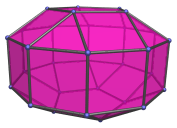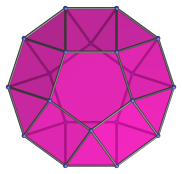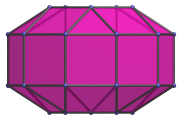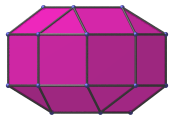# The Elongated Pentagonal Gyrobicupola

The elongated pentagonal gyrobicupola is the 39th Johnson solid (J39). It has 30 vertices, 60 edges, and 32 faces (10 equilateral triangles, 20 squares, 2 pentagons).The elongated pentagonal gyrobicupola can be constructed by attaching two pentagonal cupolae to a decagonal prism, or equivalently, inserting a decagonal prism between the two halves of the pentagonal gyrobicupola (J31). The gyro- in the name refers to how the top and bottom pentagons are rotated with respect to each other. If they are aligned with each other instead, the elongated pentagonal orthobicupola (J38) is produced instead.

## Projections

Here are some views of the elongated pentagonal gyrobicupola from various angles:

Projection DescriptionTop view.Front view.Side view.

## Coordinates

The Cartesian coordinates of the elongated pentagonal gyrobicupola with edge length 2 are:

• (0, √((10+2√5)/5), 1+2√((3−φ)/5))
• (±φ, √((5−√5)/10), 1+2√((3−φ)/5))
• (±1, −√((5+2√5)/5), 1+2√((3−φ)/5))
• (±2φ, 0, ±1)
• (±1, ±√(3+4φ), ±1)
• (±φ2, ±√(2+φ), ±1)
• (0, −√((10+2√5)/5), −(1+2√((3−φ)/5)))
• (±φ, −√((5−√5)/10), −(1+2√((3−φ)/5)))
• (±1, √((5+2√5)/5), −(1+2√((3−φ)/5)))

where φ=(1+√5)/2 is the Golden Ratio.

Last updated 03 Sep 2018.